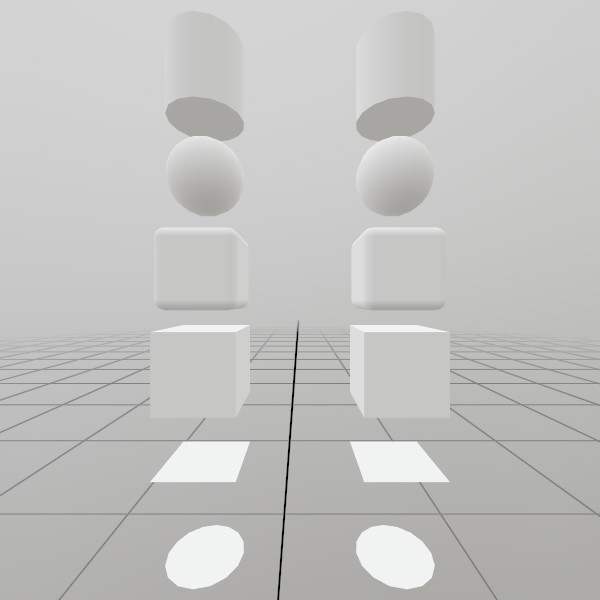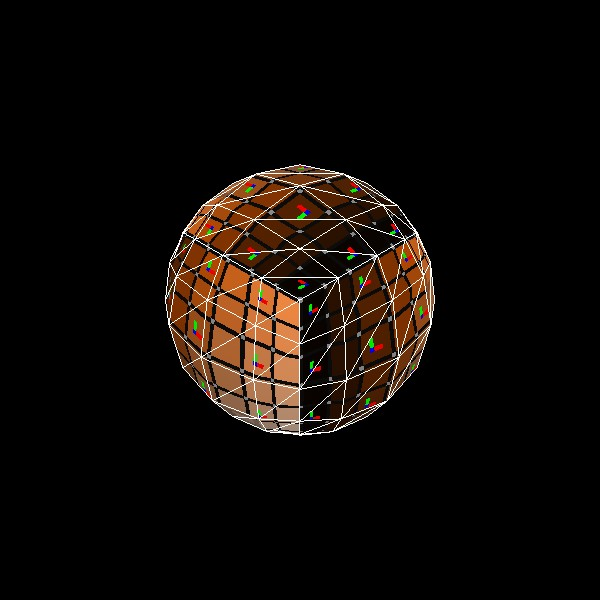# Mesh.GenerateSphere

``````static Mesh GenerateSphere(float diameter, int subdivisions)
``````

Generates a sphere mesh, pre-sized to the given diameter, created by sphereifying a subdivided cube! UV coordinates are taken from the initial unspherified cube.

NOTE: This generates a completely new Mesh asset on the GPU, and is best done during ‘initialization’ of your app/scene. You may also be interested in using the pre-generated `Mesh.Sphere` asset if it already meets your needs.

float diameter The diameter of the sphere in meters, or 2*radius. This is the full length from one side to the other.
int subdivisions How many times should the initial cube be subdivided?
RETURNS: Mesh A sphere mesh, pre-sized to the given diameter, created by sphereifying a subdivided cube! UV coordinates are taken from the initial unspherified cube.

## Examples

### Generating a Mesh and ModelHere’s a quick example of generating a mesh! You can store it in just a Mesh, or you can attach it to a Model for easier rendering later on.

``````// Do this in your initialization
Mesh  sphereMesh  = Mesh.GenerateSphere(0.4f);
Model sphereModel = Model.FromMesh(sphereMesh, Default.Material);
``````

Drawing both a Mesh and a Model generated this way is reasonably simple, here’s a short example! For the Mesh, you’ll need to create your own material, we just loaded up the default Material here.

``````// Call this code every Step

Matrix sphereTransform = Matrix.T(-.5f, .5f, 0);
sphereMesh.Draw(Default.Material, sphereTransform);

sphereTransform = Matrix.T(.5f, .5f, 0);
sphereModel.Draw(sphereTransform);
``````

### UV and Face layout

Here’s a test image that illustrates how this mesh’s geometry is laid out.``````meshSphere = Mesh.GenerateSphere(1);
``````

Found an issue with these docs, or have some additional questions? Create an Issue on Github!